Jarvis' Blog 总有美丽的风景让人流连

# Convolution Arithmetic for Deep Learning

2019-06-06
2020-10-28
Jarvis
Post

## 第1章 引言

### 1.1 离散卷积(discrete convolution)

$\mathbf{y = Wx + b}. \label{eq:affine}$

1. 多属性的数据: 每个数据点包含 $n$ 个属性值, 形成一个 $n$ 维向量. 不同数据点的特征向量长度相同. 比如一个鸢尾花(iris)的数据点包含的五个属性(种属, 萼片长度, 萼片宽度, 花瓣长度, 花瓣宽度)构成了一个五维特征向量.
2. 图像: 每幅图像看作一个数据点, 图像的高度和宽度为 $h$ 和 $w$, 包含 $h\times w$ 个属性值, 形成一个二维矩阵. 不同数据点的长宽可能均不同. 图像的两个维度称为空间维度.
3. 时序数据: 每段时序数据看作一个数据点, 每个时间点包含 $n$ 个属性, 时序长度为 $t$, 形成一个二维矩阵. 不同数据点的属性维度相同, 时序长度不同. 时序数据的数据点也是二维矩阵, 一维表示属性, 一维表示时间序列. 比如一段气象数据为一个数据点, 包含五种属性(温度, 湿度, 气压, 风速, 风向), 30分钟为一个时间序列, 每分钟采样依次, 则构成 $5\times 30$ 的二维矩阵.

$(f\star g)(t) = \int_{-\infty}^{\infty}f(\tau)g(t-\tau)d\tau.$

$(f\star g)(n) = \sum_{m=-\infty}^{\infty}f(m)g(n-m).$

$g(\tau)=\sqrt{r^2-\tau^2},\;\tau\in[-r, r]$
$f\star g=\int_{-\infty}^{\infty}f(\tau)g(t-\tau)d\tau$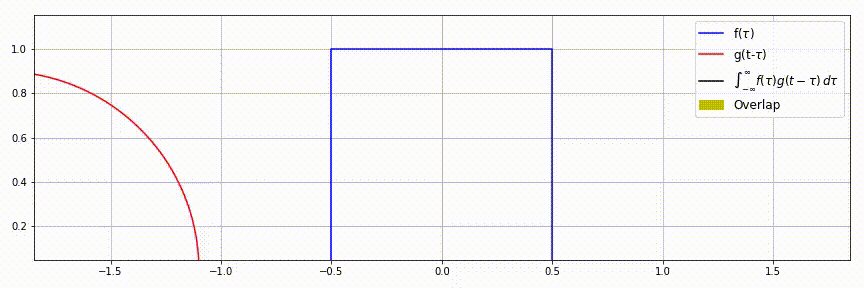$g(m)=w_m, m\in\{0, 1, 2\}$
$(f\star g)(n)$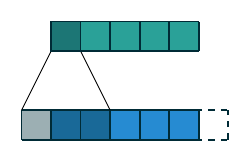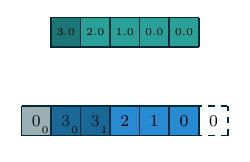$g(m, n)=w_{m, n}, m, n\in\{0, 1, 2\}$
$(f\star g)(n)$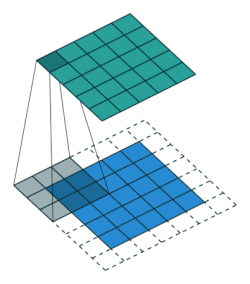• 输入的特征图: 上表中的蓝色区域(仅显示了一个通道), 一般来说 shape=[h_in, w_in, c_in]
• 卷积核: 上表中的阴影区域(仅显示了一个卷积核的一个通道), 一般来说 shape=[k, k, c_in, c_out]
• 输出的特征图: 上表中的青色区域(仅显示了一个通道), 一般来说 shape=[h_out, w_out, c_out]

• $i_j$ 输入特征图沿第 $j$ 轴的尺寸(input)
• $k_j$ 卷积核沿第 $j$ 轴的尺寸(kernel)
• $s_j$ 卷积操作沿第 $j$ 轴的步长(stride)
• $p_j$ 沿第 $j$ 轴首和尾填充零的个数(padding)
• $r_j$ 膨胀卷积的膨胀率(rate)
• $o_j$ 输入特征图沿第 $j$ 轴的尺寸(output)

## 第2章 卷积运算

### 2.1 卷积

$o=(i-k)+1$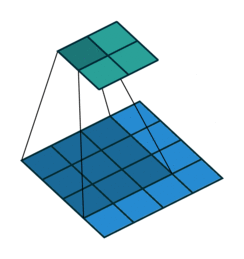$o=(i-k)+2p+1$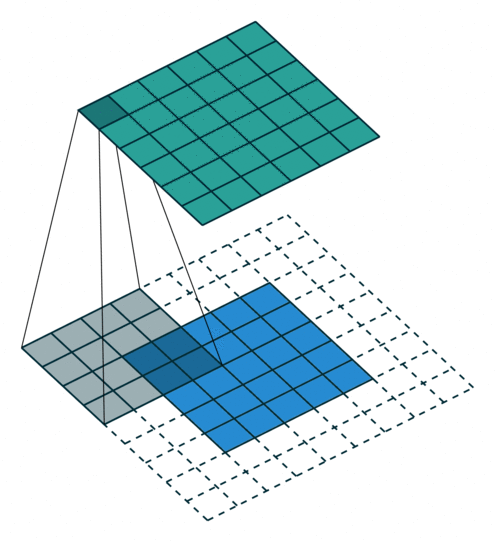same, $o=i$ $p=\left\lfloor k/2\right\rfloor, s=1$
$o=i$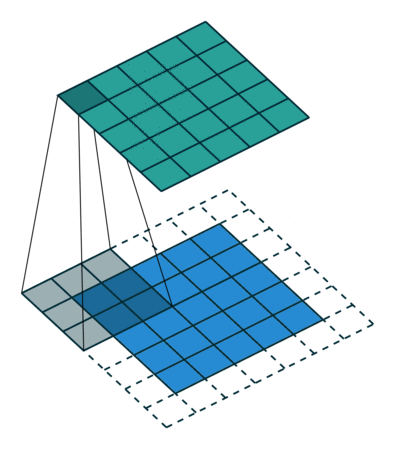$o=i+(k-1)$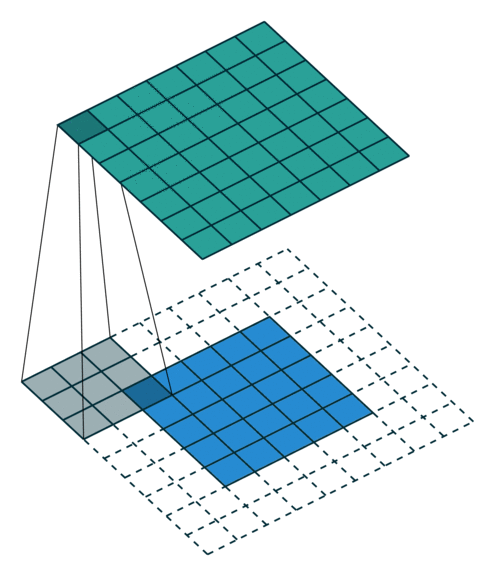$\displaystyle o=\left\lfloor\frac{i-k}{s}\right\rfloor+1$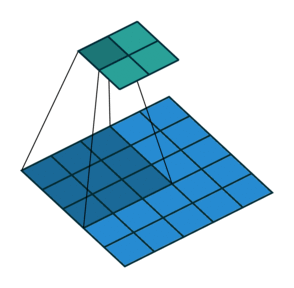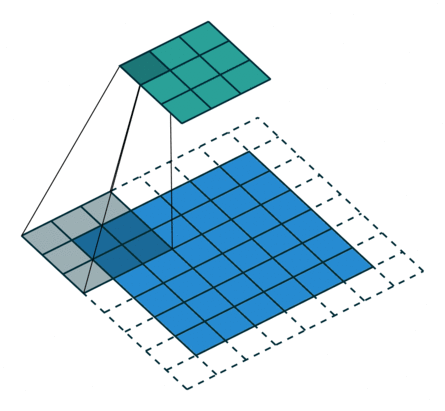### 2.2 转置卷积

$\mathbf{x} = \mathbf{A}^T\mathbf{y},\quad y\in\mathbb{R}^n, x\in\mathbb{R}^{2n}.$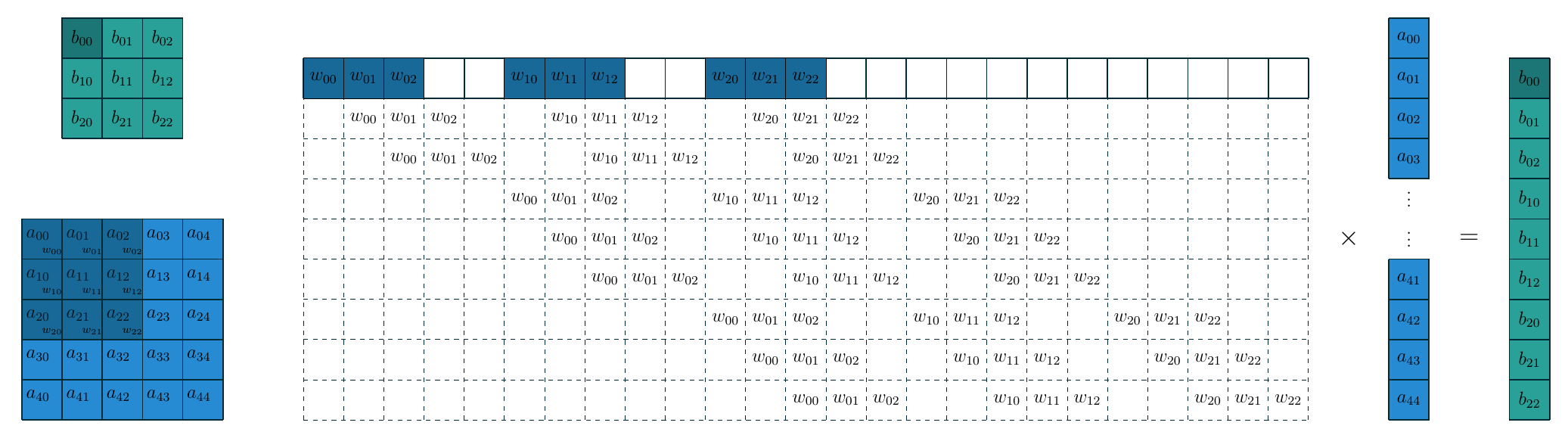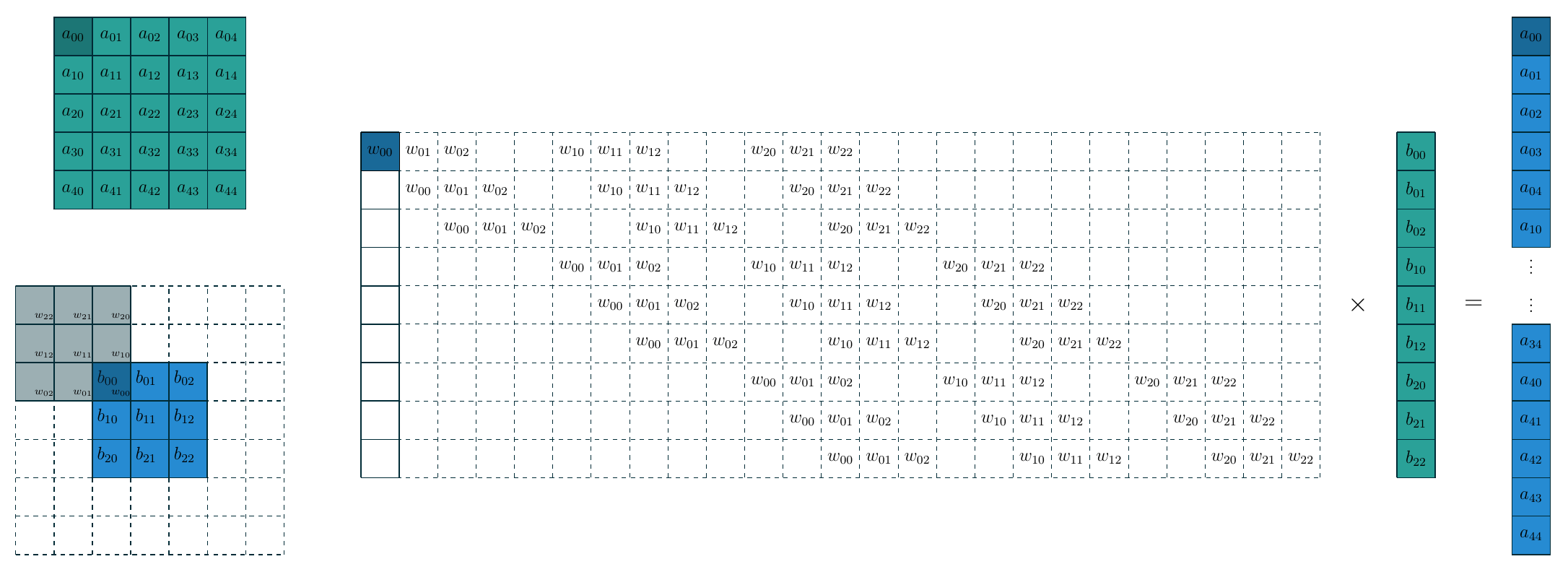### 2.3 再看卷积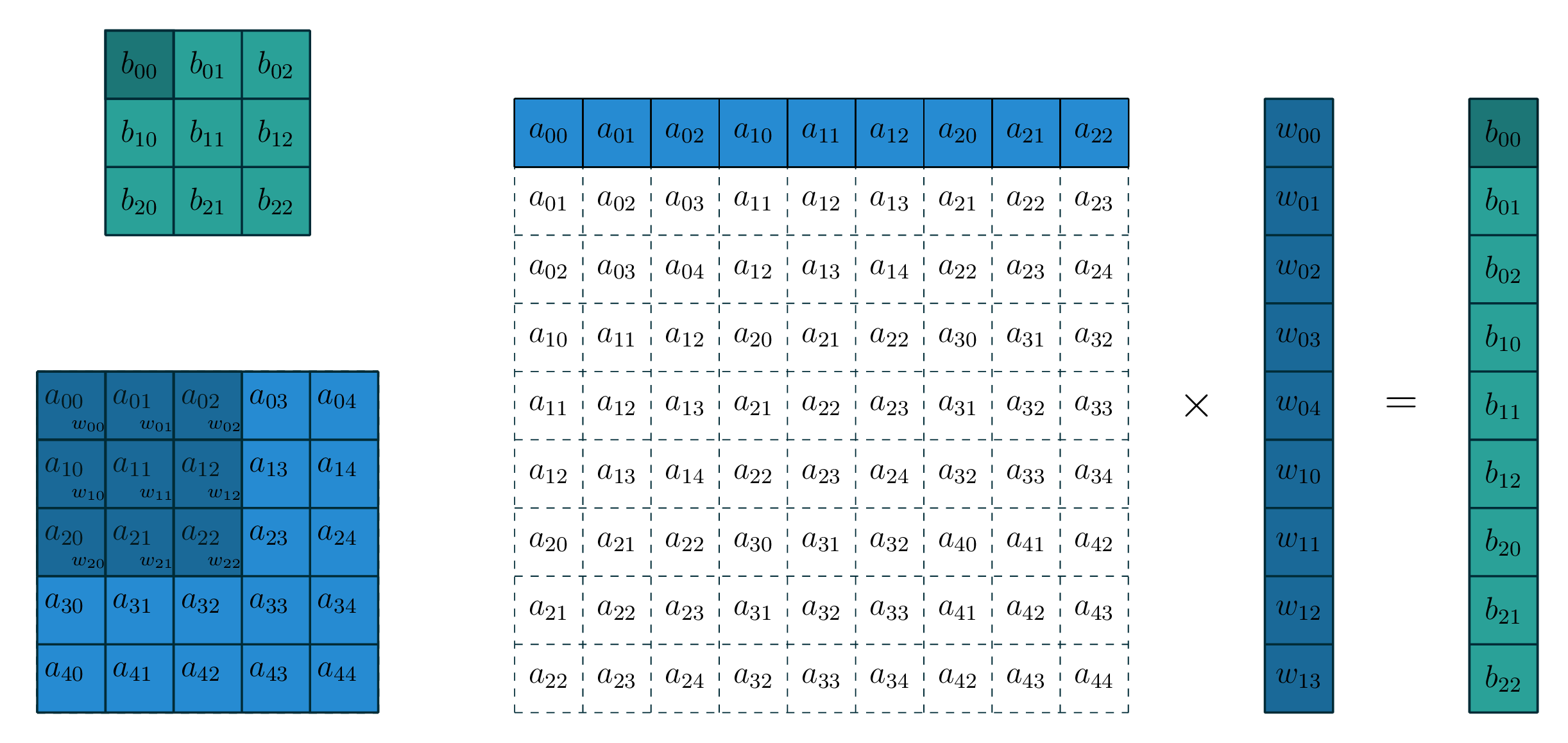## 动图生成

1
2
3
4
5
6
7
8
9
10
11
12
13
14
15
16
17
18
19
20
21
22
# 在 Ubuntu 下, 预先安装 apt install ImageMagick 用于格式转换
# 测试安装是否成功
# convert -h
# 安装 Latex 软件, 这里的代码需要 pdflatex 引擎

# 进入项目目录
cd jarvis73.github.io/code/conv_arithmetic

# 1. 首先生成逐帧的pdf
# 卷积
# python ./code/20190606-Conv.py conv
# 反卷积
# python ./code/20190606-Conv.py conv_transpose
# 另一种卷积的展开方式(框架里的用法)
python ./20190606-Conv.py conv_v2

# 2. pdf 转 png
bash ./pdf2png.sh 201906_conv_v2

# 3. 合并 png 为 gif
convert -delay 60 201906_conv_v2_png/*.png output.gif



## 参考文献

1. Gradient-based learning applied to document recognition
Yann LeCun, Lon Bottou, Yoshua Bengio, Patrick Haffner.
[link]. In Proceedings of the IEEE[J], 1998, 86(11): 2278-2324.

2. Imagenet classification with deep convolutional neural networks
Krizhevsky A, Sutskever I, Hinton G E.